# Electric Field of a Uniform Ring of Charge

• ChiralSuperfields
Thanks for your reply! I am very sorry kuruman, I used the wrong terminology for the integrals. I was actually asking why they don't have limits of integration. I think they don't because they already know that the sum of charge elements dq across the ring was equal to Q. Many...

#### ChiralSuperfields

Homework Statement
A ring of radius a carries a uniformly distributed positive total charge Q. Calculate the electric field due to the ring at a
point P lying a distance x from its center along the central axis perpendicular to the plane of the ring (Fig. 23.3a).
Relevant Equations
continuous charge distribution formula
Hi!

For this problem,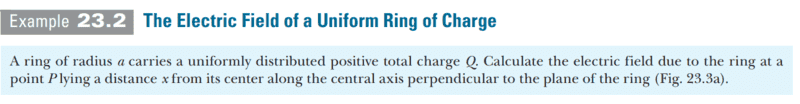The solution is,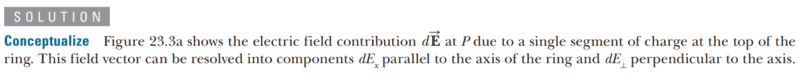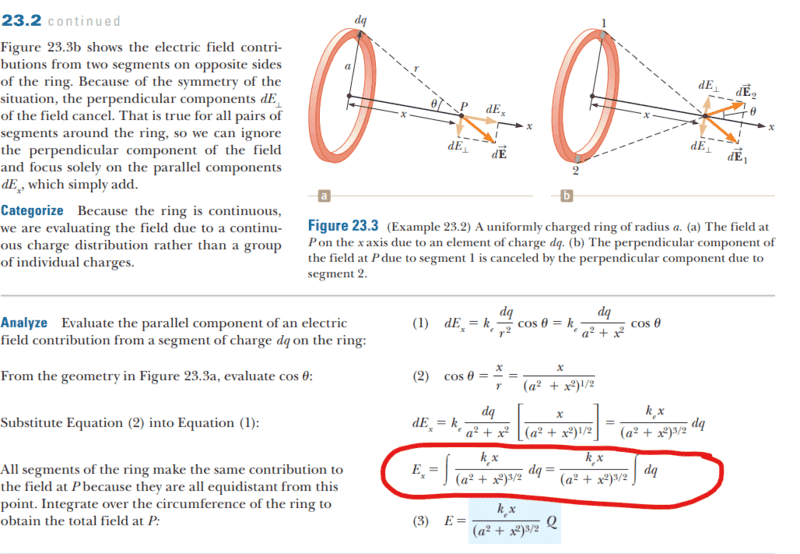However, why did they not include constants of integration in their working shown in red?

Many thanks!

Callumnc1 said:
Homework Statement:: A ring of radius a carries a uniformly distributed positive total charge Q. Calculate the electric field due to the ring at a
point P lying a distance x from its center along the central axis perpendicular to the plane of the ring (Fig. 23.3a).
Relevant Equations:: continuous charge distribution formula

Hi!

For this problem,
View attachment 317980
The solution is,
View attachment 317981
View attachment 317982
However, why did they not include constants of integration in their working shown in red?

Many thanks!
No constants of integration are called for in this case. The integral ##\int dq## is shorthand for "Add all the infinitesimally small elements ##dq## into which total charge ##Q## has been divided."

What do you get when you do that?
What else? The total charge on the ring ##Q##.

•ChiralSuperfields
kuruman said:
No constants of integration are called for in this case. The integral ##\int dq## is shorthand for "Subdivide the total charge on the ring, ##Q##, into many small elements ##dq## and add them all up."

What do you get when you do that?
What else? The total charge on the ring ##Q##.
Thanks for your reply! I see what you are getting at now. All the small charge elements dq across the ring add up to Q.

However, why do they not have the limits of integration. I think they don't because the already know that the sum of charge elements dq across the ring was equal to Q.

Many thanks!

Last edited:
My personal preference is not to use constants of integration in physics problems and use instead upper and lower limits of integration. Consider, for example, the simple question of finding the velocity as a function of time for an object that moves under constant acceleration. One has to integrate both sides of the equation ##dv=a~dt ## where ##a## is constant. One might write the mathematically correct expression that is taught in intro calculus in terms of a constant of integration,$$v=at+C.$$ Because the equation involves physical entities associated with the physical motion of an object, I view the integration as adding up infinitesimal elements of velocity on the LHS and acceleration times elements of time on the RHS. As with any addition, you have to start and end somewhere and that's where the limits of integration come in. I start the addition when the clock timing the motion reads ##t_0##, at which time the velocity is ##v_0##, and stop adding when the clock reds ##t## and the velocity is ##v##. This is addition is written symbolically as $$\int_{v_0}^{v}dv=a\int_{t_0}^{t}dt$$ from which $$v-v_0 = a(t-t_0).$$ With ##t_0=0##, which is usually the case, and after some rearrangement one gets the familiar equation $$v=v_0+at.$$ The integration constant aficionados will correctly tell you that this is the same as setting the integration constant ##C=v_0##. I prefer using definite integrals because it reminds me that integration is nothing but addition and because any needed integration constant is taken into account automatically.

•BvU and ChiralSuperfields
kuruman said:
My personal preference is not to use constants of integration in physics problems and use instead upper and lower limits of integration. Consider, for example, the simple question of finding the velocity as a function of time for an object that moves under constant acceleration. One has to integrate both sides of the equation ##dv=a~dt ## where ##a## is constant. One might write the mathematically correct expression that is taught in intro calculus in terms of a constant of integration,$$v=at+C.$$ Because the equation involves physical entities associated with the physical motion of an object, I view the integration as adding up infinitesimal elements of velocity on the LHS and acceleration times elements of time on the RHS. As with any addition, you have to start and end somewhere and that's where the limits of integration come in. I start the addition when the clock timing the motion reads ##t_0##, at which time the velocity is ##v_0##, and stop adding when the clock reds ##t## and the velocity is ##v##. This is addition is written symbolically as $$\int_{v_0}^{v}dv=a\int_{t_0}^{t}dt$$ from which $$v-v_0 = a(t-t_0).$$ With ##t_0=0##, which is usually the case, and after some rearrangement one gets the familiar equation $$v=v_0+at.$$ The integration constant aficionados will correctly tell you that this is the same as setting the integration constant ##C=v_0##. I prefer using definite integrals because it reminds me that integration is nothing but addition and because any needed integration constant is taken into account automatically.
Thanks for your reply! I am very sorry kuruman, I used the wrong terminology for the integrals. I was actually asking why they don't have limits of integration. I think they don't because they already know that the sum of charge elements dq across the ring was equal to Q. Many thanks!

This is a path integral where the path is a closed curve. If you integrate the path element you get the length of the curve. With no extra constant. So, with a linear charge density ##\lambda##, you can write ##dq=\lambda ds## and the integral of ##dq## will be actually ##\lambda \int{ds}## where ##\int{ds}## is the length of the path, in this case, the full circle. You can skip all this if you realize that the integral of dq is just the total charge.

•ChiralSuperfields
nasu said:
This is a path integral where the path is a closed curve. If you integrate the path element you get the length of the curve. With no extra constant. So, with a linear charge density ##\lambda##, you can write ##dq=\lambda ds## and the integral of ##dq## will be actually ##\lambda \int{ds}## where ##\int{ds}## is the length of the path, in this case, the full circle. You can skip all this if you realize that the integral of dq is just the total charge.
Thank you nasu!

Apparently, this is a 'contour integral':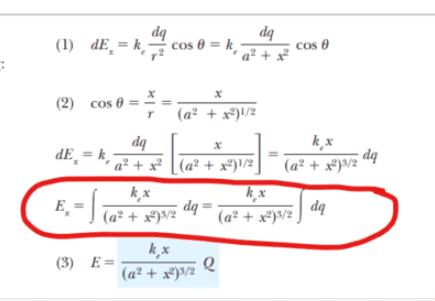So the start point is equal to the end point, so it has no limits? But if the start point is equal to the end point then could we not just have the limits to be anything as long as they are the same? For example, could we have upper and lower limits to be Q, however, by the second fundamental theorem of calculus, they cancel!?

I guess if you have the same limits then the area under the curve is zero.

Many thanks!

Callumnc1 said:
Apparently, this is a 'contour integral':
View attachment 318039
So the start point is equal to the end point, so it has no limits? But if the start point is equal to the end point then could we not just have the limits to be anything as long as they are the same? For example, could we have upper and lower limits to be Q, however, by the second fundamental theorem of calculus, they cancel!?

I guess if you have the same limits then the area under the curve is zero.

Many thanks!
This is an area integral. You are integrating over the area of the ring.

•nasu
String theory guy said:
This is an area integral. You are integrating over the area of the ring.
Thank for you reply @String theory guy ! However, I would like to understand how to solve this problem using limits of integration. I understand that we are integrating over the of the ring, so the start point will be the same as the end point.

However, does this mean that the lower and upper limits of integration will be the same. From the second fundamental theorem of calculus this does not make sense because it will cancel.

Many thanks!

Last edited:
String theory guy said:
This is an area integral. You are integrating over the area of the ring.
This is not an area integral. Look at Figure 23.3 in post #1. What is the area over which the integral is taken?

•ChiralSuperfields
kuruman said:
This is not an area integral. Look at Figure 23.3 in post #1. What is the area over which the integral is taken?
Yep thanks for pointing that out - I meant to say line integral @kuruman .

•ChiralSuperfields
String theory guy said:
Yep thanks for pointing that out - I meant to say line integral @kuruman .
Sorry, but it's not even a line integral. Line integrals have the form ##I=\int \mathbf{F}\cdot d\mathbf{s}##, where ##\mathbf{F}## is a vector at line element ##d\mathbf{s}.## An example of a line integral is the work done by a force ##\mathbf{F}## on an object that moves from point A to point B. $$W_{AB}=\int_A^B \mathbf{F}\cdot d\mathbf{s}.$$It is not my intention to be didactic; it's important to be on the same page about what we mean and what we understand to be the case.

••ChiralSuperfields and String theory guy
kuruman said:
Sorry, but it's not even a line integral. Line integrals have the form ##I=\int \mathbf{F}\cdot d\mathbf{s}##, where ##\mathbf{F}## is a vector at line element ##d\mathbf{s}.## An example of a line integral is the work done by a force ##\mathbf{F}## on an object that moves from point A to point B. $$W_{AB}=\int_A^B \mathbf{F}\cdot d\mathbf{s}.$$It is not my intention to be didactic; it's important to be on the same page about what we mean and what we understand to be the case.
No worries, I should of been more specific. It is a line charge integral @kuruman .

•ChiralSuperfields
nasu said:
This is a path integral where the path is a closed curve. If you integrate the path element you get the length of the curve. With no extra constant. So, with a linear charge density ##\lambda##, you can write ##dq=\lambda ds## and the integral of ##dq## will be actually ##\lambda \int{ds}## where ##\int{ds}## is the length of the path, in this case, the full circle. You can skip all this if you realize that the integral of dq is just the total charge.
Sorry @nasu, could you please explain what is a path integral? They don't seem to mention that in the videos I am watching from MIT's 8.02 course. They just talk about integrating to find the field of a line charge.

Many thanks!

•ChiralSuperfields
nasu said:
It is also called line integral. You can look it up. I mean the first definition in this link: https://en.m.wikipedia.org/wiki/Path_integral
Thanks for your reply @nasu! Sorry, how is this a line integral? Don't line integrals have the form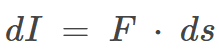where I is a scalar product of the F and ds vectors as @kuruman said in post #13? I'm confused because @String theory guy also said this was a line integral.

But I don't see how this electric field integral can be of the form of a line integral since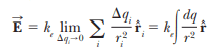Many thanks,
Callum

I already told you. You can write dq as ##\lambda ds ##. And actually everything but the ##ds## term is a constant and goes outside the integral.

•ChiralSuperfields
nasu said:
I already told you. You can write dq as ##\lambda ds ##.
Thanks for your reply @nasu! Sorry, how is this a line integral? (That is my confusion now)

And my confusion is about what do you mean by "how".

•ChiralSuperfields
nasu said:
And my confusion is about what do you mean by "how".
Thanks for your reply @nasu! I mean by 'how' is that I though line integrals were of the form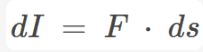. However, I'm confused because I don't see any dot product in the electric field formulae. Many thanks!

Who said that it has to be a dot product? The work of a force is an example of line integral but it is not the only case. See here, for example:
https://en.wikipedia.org/wiki/Line_integral
You integrate a function along a curve. The function can be a vector or a scalar.

•ChiralSuperfields
nasu said:
Who said that it has to be a dot product? The work of a force is an example of line integral but it is not the only case. See here, for example:
https://en.wikipedia.org/wiki/Line_integral
You integrate a function along a curve. The function can be a vector or a scalar.
Thanks for your reply @nasu! Is that the most general definition of a line integral? A function that is getting to integrate along a curve.

I'm then guessing that the most general definition of an area (or surface) integral is a function that is getting integrated over a surface.

And for a volume integral, a function that is getting integrated over a volume.

Many thanks!

Sorry, I am not a mathematician to worry about the most general definitions.

•ChiralSuperfields
nasu said:
Sorry, I am not a mathematician to worry about the most general definitions.
Thanks for your reply @nasu! It's interesting how you call what is being integrated as the 'function'. That means that the function in this case is 1 (1 is inside the dq integral). So, 1 is getting integrated over the line to become Q.

My question is now, what is 1 a function of inside the integral?

Many thanks!

Do you have a problem with constant functions in general or just when the constant is 1? What is the point of asking this question for a constant function? I guess you can say it is a function defined on the domain of integration, at least. But how will this change anything? If you have one of the "normal" integrals like ##\int dx ## do you have a problem with what is 1 a function of?

•ChiralSuperfields
Thanks for your reply @nasu! How would 1 (or any other constant) be a function? I thought they had to be a function of some variable.

Many thanks,
Callum

https://en.wikipedia.org/wiki/Constant_function
I am not sure what you are trying to learn but I feel that you are asking irrelevant questions. At least for physics.Maybe you should start by studying systematically some algebra and basic analysis so you become familiar with some, well, basic things. Constant functions are a quite trivial thing in this context.

•ChiralSuperfields
nasu said:
https://en.wikipedia.org/wiki/Constant_function
I am not sure what you are trying to learn but I feel that you are asking irrelevant questions. At least for physics.Maybe you should start by studying systematically some algebra and basic analysis so you become familiar with some, well, basic things. Constant functions are a quite trivial thing in this context.
Thank you for your help @nasu and sorry for the irreverent math questions. I am meant to be taking a real analysis, algebra and multivariate math courses next year, so that should sort it outMany thanks!